# Year 10+ Plane Geometry

## The Triangle Proportionality Theorem

This theorem states that if $ADE$ is a triangle, and $BC$ is drawn parallel to the side $DE$, then $\dfrac{AB}{BD} = \dfrac{AC}{CE}$.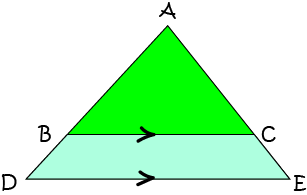Proof: To prove this theorem (show it is true), we add an extra line $BF$ parallel to $AE$ to form parallelogram $BCEF$ as shown in the diagram below: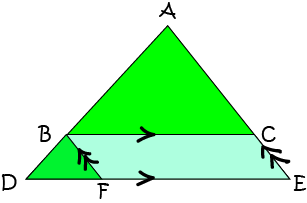Next, we show that triangles $ABC$ and $BDF$ are similar:

• $\angle ABC = \angle BDF$ as they are corresponding angles.
• $\angle BFD = \angle ACB$ as they are alternate (interior) angles.
Therefore, the two triangles are similar as they are equiangular.

We use corresponding sides of similar triangles $ABC$ and $BDF$ to establish the result:

• Sides $AB$ and $BD$, and sides $AC$ and $BF$ are corresponding sides.
• Since the triangles are similar, $\dfrac{AB}{BD} = \dfrac{AC}{BF}$.
• $BF = CE$ (opposite sides of parallelogram $BCEF$).
• So, $\dfrac{AB}{BD} = \dfrac{AC}{CE}$, establishing our result.

### Example 1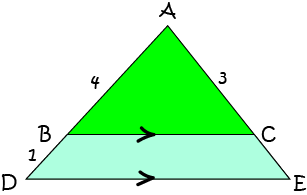Find the length of $CE$.

Solution:

By the Triangle Proportionality Theorem, $\dfrac{AB}{BD} = \dfrac{AC}{CE}$. So,

\begin{align*} \dfrac{4}{1} &= \dfrac{3}{CE}\\ CE &= \dfrac{3}{4} \end{align*}

## The Angle Bisector Theorem

This theorem states that if $ABC$ is a triangle, and $AD$ is drawn so that it bisects $\angle BAC$, then $\dfrac{AB}{BD} = \dfrac{AC}{DC}$.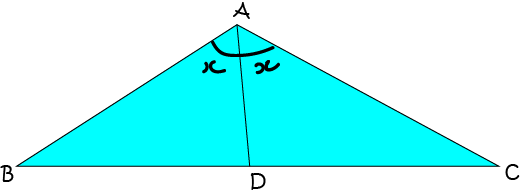Proof: To prove this theorem (show it is true), we label the triangle as as shown in the diagram below: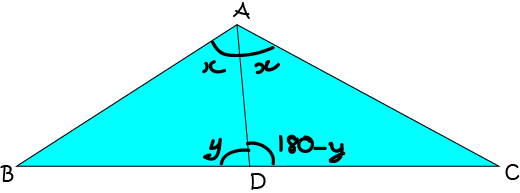Then

• $\angle BAD = \angle DAC = x^\circ$ as $AD$ bisects $\angle BAC$.
• and we let $\angle ADB = y^\circ$, so, as they are angles on the same straight line, $\angle ADC = (180 - y)^\circ$.
• Using the Sine Law on $\triangle ACD$ gives

$\dfrac{\sin x^\circ}{BD} = \dfrac{\sin y^\circ}{AB}$.
Rearranging yields
$\dfrac{AB}{BD} = \dfrac{\sin y^\circ}{\sin x^\circ}$.
Similarly, applying the Sine Law to $\triangle ACD$ gives
$\dfrac{AC}{DC} = \dfrac{\sin (180 - y)^\circ}{\sin x^\circ}$.

The result follows since $\sin (180 - y)^\circ = \sin y^\circ$. Therefore,

$\dfrac{AC}{DC} = \dfrac{\sin y^\circ}{\sin x^\circ} = \dfrac{AB}{BD}.$

An interesting consequence of this result is that if $\triangle ABC$ is isosceles, then triangles $ABD$ and $ACD$ are congruent.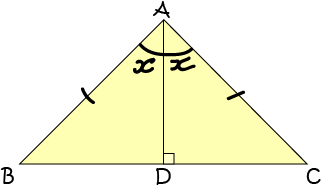### Example 2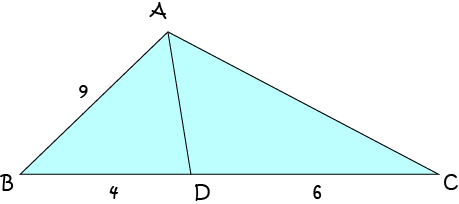Find the length of $AC$.

Solution:

By the Angle Bisector Theorem, $\dfrac{AB}{BD} = \dfrac{AC}{DC}$. So,

\begin{align*} \dfrac{9}{4} &= \dfrac{AC}{6}\\ AC&= \dfrac{54}{4} = 13.5 \end{align*}

## Areas of Similar Triangles

If triangles $ABC$ and $XYZ$ are similar and have sides in the ratio $p:q$, then their areas are in the ratio $p^2:q^2$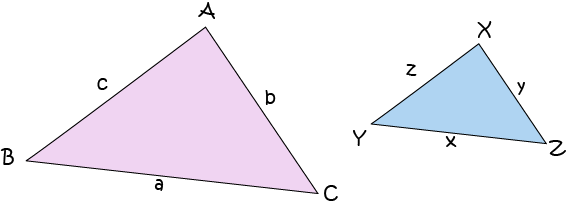Proof

Suppose triangles $ABC$ and $XYZ$ are similar and have sides in the ratio $p:q$. Then we can use the general formula for the area of a triangle to find

• $\text{Area}_{ABC} = \dfrac{1}{2}\;bc \sin A$
• $\text{Area}_{XYZ} = \dfrac{1}{2}\;yz \sin X$
Since these lengths are in the ratio $p:q$,
$\dfrac{y}{b} = dfrac{p}{q}$ and $\dfrac{z}{c} = dfrac{p}{q}$.

So, $y = \dfrac{bp}{q}$ and $z = \dfrac{cp}{q}$.

We also have $A = X$ since the triangles are similar.

So, we can calculate

\begin{align*} \text{Area}_{XYZ} &= \dfrac{1}{2}\;yz \sin X\\ &= \dfrac{1}{2}\;\left(\dfrac{bp}{q}\right) \left(\dfrac{cp}{q}\right) \sin X\\ &= \dfrac{1}{2} \; \dfrac{p^2}{q^2} bc \sin A\\ &= \dfrac{p^2}{q^2} \text{Area}_{ABC} \end{align*}
So, the areas of the two triangles are in the ratio:
$\text{Area of triangle }ABC: \text{Area of triangle }XYZ = p^2:q^2,$
as required.

### Example 3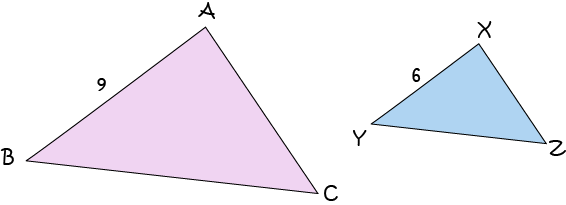The area of triangle $ABC$ is $60 \text{ cm}^2$. If triangles $ABC$ and $XYZ$ are similar, what is the area of triangle $XYZ$?

Solution:

The two triangles are similar, with corresponding sides in the ratio $6: 9$, so their areas are in the ratio $6^2:9^2 = 36:81 = 4:9$. Therefore, the area of triangle $XYZ$ is equal to

$\dfrac{4}{9}\text{Area}_{ABC} = \dfrac{4}{9} (60) = 26.6666\dots \text{ cm}^2.$

### Description

In these chapters you will learn about plane geometry topics such as

• Area (Irregular polygons, plane shapes etc)
• Perimeter
• Conic sections (Circle, Ellipse, Hyperbola etc)
• Polygons (Congruent, polygons, similar, triangles etc)
• Transformations and symmetry (Reflection, symmetry, transformations etc)

etc

Even though these chapters are marked for Year 10 or higher students, several topics are for students in Year 8 or higher

### Audience

Year 10 or higher, suitable for Year 8 + students as well.

### Learning Objectives

You must be logged in as Student to ask a Question.